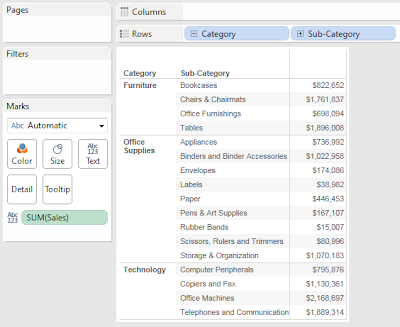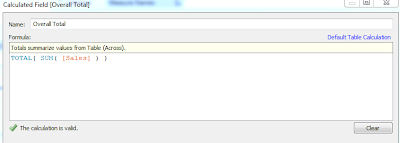## Thursday, March 21, 2013

### Working with Window Calculations and TOTAL() in Tableau

Today, we will talk about Window Calculations and the TOTAL() function in Tableau.  Window Calculations allow you to implement a second level of aggregation on top of your basic aggregations, such as SUM() and COUNT().  This post is the ancestor to Working with Table Calculations in Tableau.  If you are not familiar with Table Calculation and "Compute Using", you should read that post first.  This is also the first post where I am using Tableau 8.0.  As usual, we will use the Superstore Sales sample data set in Tableau.

First, let's look at the TOTAL() function.  The TOTAL() function applies the underlying aggregation to the entire pane instead of each individual row.

Step 1:
• Create a text table with two hierarchical dimensions on the rowsSales by Sub-Category
Step 2:
• Calculate the total sales across all categories
• Calculate the total sales for each category
• Create the following calculated fieldsCategory TotalOverall Total
Take care to notice what values are being summarized over.

Step 3:
• Add these values to the tableSales by Sub-Category (with Totals)
This example is somewhat silly because this is much better accomplished by adding Row Subtotals.  However, this is a great example to show how the TOTAL() function works.

Now, let's move on to Window Calculations.  We will use WINDOW_SUM(); however, the same techniques can be applied for the other Window Calculations as well.  A Window Calculation requires three input parameters.  These parameters are an aggregated value, a displacement value for the starting point, and a displacement value for the ending point.  For example,

WINDOW_SUM( SUM( [Sales] ), 0, 0 )

will calculate the SUM of SUM of [Sales] for the current row only, which is just the SUM of [Sales]; whereas

WINDOW_SUM( SUM( [Sales] ), FIRST(), LAST() ) or just WINDOW_SUM( SUM( [Sales] ) )

will calculate the SUM of SUM of [Sales] for the entire pane, which is identical to using

TOTAL( SUM( [Sales] ) ).

Step 1:
• Create a text tableSales by Sub-Category
Step 2:
• Calculate the sum of each Sub-Category along with the previous and next Sub-Category
• Create the following calculated fieldSum of Neighbors
Step 3:
• Add this value to the tableSales by Sub-Category (with Sum of Neighbors)
Now, you can see that each value is the Sum of [Sales] for this Sub-Category as well as the two neighboring Sub-Categories.  Care should be taken to avoid situations such as the first as last row, where there is no previous or next value, respectively.

There is so much more to be done here.  Try combining Window Calculations with different aggregrations, such as Sums of Averages, or try creating your own Percent of Total calculations.  I hope you found this informative.  Thanks for reading.

Associate Consultant
Mariner, LLC
llewellyn.wb@gmail.com

1.Hi

This looks like a great approach - however when i duplicate the effort with multiple extra dimension the figures does no longer summarize per category but per line only.

Have you any experience with this?

Christian

2.I replicate this and got the same results but many of the sum of neighbors are correct. Ex. Copiers, Office Machines, Telephones are correct. Whereas Chairs, Tables, Appliances are incorrect.

3.I donthave the "container" dimension in my excel , which excel can i get it.

4.Hi Brad, nice post. I have similar requirement help me out how to achieve. I am using tableau desktop 10.2. I have a cross tab table with different measures, percentages and derived calculations.

I have column level calculations like Col 1 = Col 12+Col11 and Col2 = Col14/Col13. When I try to add sub totals to this cross tab tableau adding all values in respective column and displaying total at the bottom. But my requirement is the subtotals should also populate values by following the column level calculations. When I work with Cognos we used to deal these things by using Solve Order functionality. Do we have similar kind of functionality to display column level calculations in sub totals. Attached is the reference doc for same.

COL 1 COL 2 COL 6 COL 7 COL 9 COL 10 COL 11 COL 12 COL 13 COL 14
Indecator 1 4.2% 6.0% 3.1% 37.5% 17,159 525 16,624 697 6,233 371
Indecator 2 2.9% 3.7% 3.1% 46.5% 15,588 477 16,111 472 7,486 276
Indecator 3 0.3% 0.3% 0.4% 28.2% 24,388 86 14,302 44 4,032 11
Indecator 4 0.2% 0.4% 0.6% 26.8% 28,728 175 28,553 67 7,651 34
Indecator Totals 1.5% 1.7% 1.47% 33.60% 85,863 1,263 75,590 1,280 25,402 692

Thanks-Vamsi.